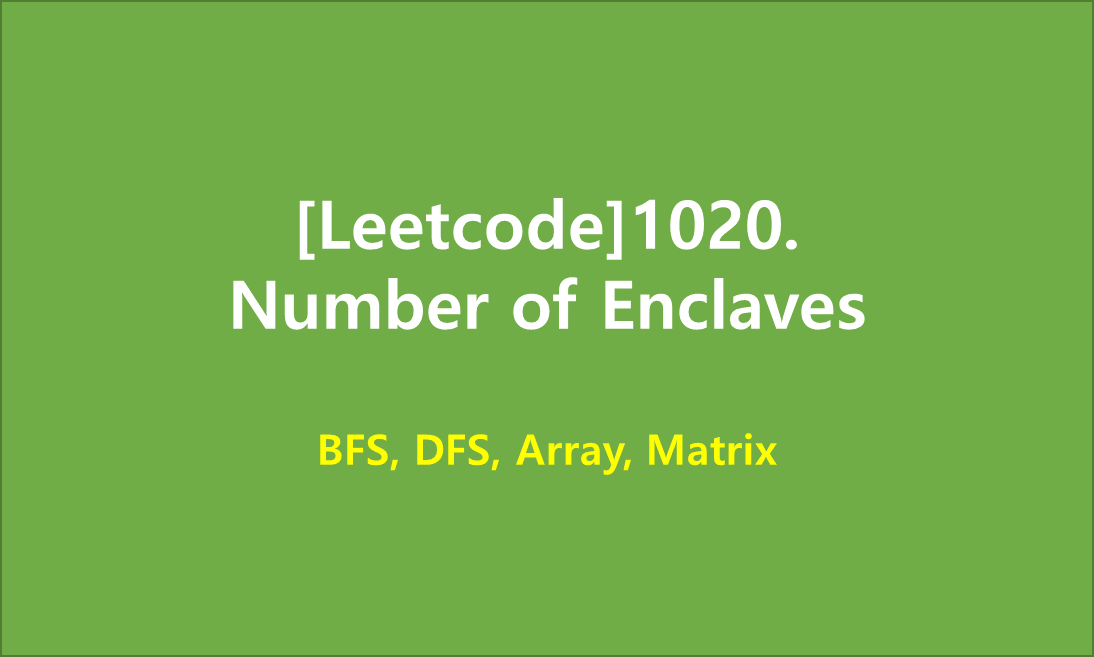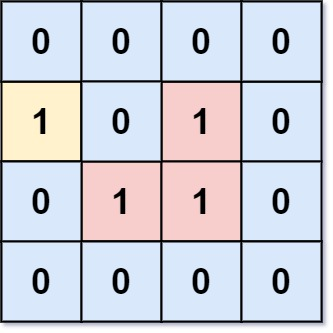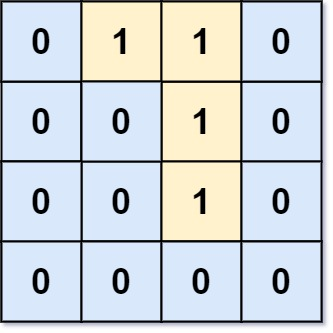# [Leetcode]1020. Number of Enclaves

limelimejiwon·2022년 5월 19일
0

## Python으로 공부하는 코딩테스트

목록 보기
61/67You are given an m x n binary matrix grid, where 0 represents a sea cell and 1 represents a land cell.

A move consists of walking from one land cell to another adjacent (4-directionally) land cell or walking off the boundary of the grid.

Return the number of land cells in grid for which we cannot walk off the boundary of the grid in any number of moves.

### Example 1:Input: grid = [[0,0,0,0],[1,0,1,0],[0,1,1,0],[0,0,0,0]]
Output: 3
Explanation: There are three 1s that are enclosed by 0s, and one 1 that is not enclosed because its on the boundary.

### Example 2:Input: grid = [[0,1,1,0],[0,0,1,0],[0,0,1,0],[0,0,0,0]]
Output: 0
Explanation: All 1s are either on the boundary or can reach the boundary.

### Constraints:

• m == grid.length
• n == grid[i].length
• 1 <= m, n <= 500
• grid[i][j] is either 0 or 1.

## 💻 My Submission

class Solution:
cnt=0
def numEnclaves(self, grid: List[List[int]]) -> int:
m, n=len(grid), len(grid)

def count(i,j, grid):
# if its boundary
if i<0 or j<0 or i>=m or j>=n:
return -(m*n)
if grid[i][j]==0:
return 0
# mark visited cell
grid[i][j]=0
# check 4-direction cell
top=count(i-1,j,grid)
bottom=count(i+1,j,grid)
left=count(i,j-1,grid)
right=count(i,j+1,grid)

return 1+ top + bottom + left + right

for i in range(m):
for j in range(n):
if grid[i][j]==1:
check=count(i,j,grid)
if check>0:
self.cnt+=check

return self.cnt

## 🎈 Another Clean Solution

• We check edges of A matrix.
• If A[i][j] is 1 on the edge, do DFS and clean all connected 1's
• Return sum of left 1's
class Solution:
def numEnclaves(self, A: List[List[int]]) -> int:
def dfs(i, j):
A[i][j] = 0
for x, y in (i - 1, j), (i + 1, j), (i, j - 1), (i, j + 1):
if 0 <= x < m and 0 <= y < n and A[x][y]:
dfs(x, y)
m, n = len(A), len(A)
for i in range(m):
for j in range(n):
if A[i][j] == 1 and (i == 0 or j == 0 or i == m - 1 or j == n - 1):
dfs(i, j)
return sum(sum(row) for row in A)

References